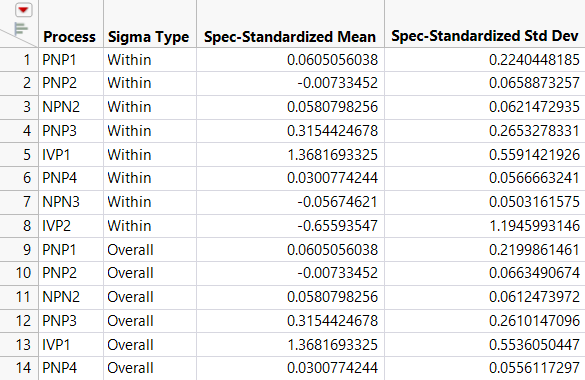Publication date: 07/30/2020

## Make Goal Plot Summary Table

The Make Goal Plot Summary Table option produces a summary data table that includes each variable’s name, its spec-normalized mean shift (Spec-Standardized Mean), and its spec-normalized standard deviation (Spec-Standardized Std Dev). For each variable, there is a row for each of the sigma types.

Note: If a variable is fit with a distribution other than normal, the name of the fitted distribution is appended parenthetically to the variable name. The Spec-Standardized Mean and Spec-Standardized Std Dev values are not provided for nonnormal variables.

The points in the Goal Plot are linked to the rows in the Goal Plot Summary Table. If you apply row states to a point in the Goal Plot, you can change the corresponding row states in the Goal Plot Summary Table. Conversely, if you apply row states in the Goal Plot Summary Table, they are reflected on the Goal Plot.

Figure 7.20 shows the Goal Plot Summary Table for the Semiconductor Capability.jmp sample data table as described in Example of the Process Capability Platform with Normal Variables.

Figure 7.20 Summary Table